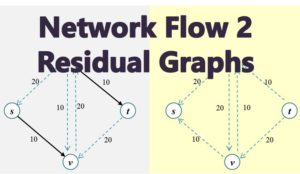# Ford-Fulkerson Algorithm for Max Flow Problem

We cover the following1. Introduction to Ford-Fulkerson Algorithm

The Ford-Fulkerson algorithm is the algorithm used to find the max flow through a network. So given a network with the capacities of the edges,  how do we assign flow to the edges until  we get the max flow?

This is how it works:

Start by assigning  a flow of 0 (f(e) = 0) to all the edges. Then check that the capacity constraint and the conservation constraint have not been violated.

Then choose particular path from s to t and increase the flow along that path. This is illustrated in Figure 1 a -f.

• From Figure 1.a, we can see the initial network with capacities for each of the edges.
• In 1b, we start by assigning a flow of 0 (f(e) = 0 to all edges
• In 1c, we choose a path (s-u-v-t) so that that we can increase flow in this path.
• In 1d, we increase the flow on all the edges on this path by 20  while leaving f(e) = 0 on other two edges (s,v) and (u,t)
• At this point, we can check if we have achieved the maximum flow through the network. The answer is no, because we have a flow of 20 but the capacity of the network is 30. So we can improve the flow.
• So in 1e, we subtract a flow of 10 from edge (u, v). This is a equivalent to adding a backward flow of 10 from on edge (v,u).
• Finally in 1f, we add a flow of 10 to edges (s, v) and (u, t).

The algorithm ends at this point and we have achieved a flow of 30, which is the maximum flow for this particular example.

There are two basic operations that was performed:

• we push a forward flow on edges with leftover capacity
• we push a backward flow on edges already carrying  a flow

2. Introduction to Residual Graphs

A residual graph is defined on a flow network G which has a flow f as Gf of G with respect to f. Gf is is defined as follows:

• The set of nodes in Gf is the same as in G
• For each edge  e = (u, v) of G, where f(e) < ce, there are ce – f(e) units which we can push forward by. So we include an edge e’ = (u, v) in Gf  with capacity ce – f(e). This is a forward edge in same direction as the original edge.
• For each edge of G,  where f(e) > 0, there are f(e) units of flow that we can ‘push backward’ or undo. So we include and edge e’ = (u, v) in  Gf  with capacity of f(e). These are in opposite direction to e and is a backward edge.

The residual graph for Figure 1 after 1d and after 1f is given in Figure 2.

Each edge in the original graph G yields one or two edges in the residual graph Gf. If 0 < f(e) < ce, then it would result in both a forward and a backward edge in the residual graph Gf. This also means that the number  of edges in the residual graph is at most twice the number of edges in the original graph G.

The capacity of edges in the residual graph is called residual capacity.

4. Augmenting Paths in a Residual Graph Using Bottleneck

Now that we have built up the residual graph, we can then proceed to examine how to analyse it. That is how to push flows from s to t in the residual graph.  Assuming that P is a simple path in Gfrom s to t which means that P does not contain any loops. The we can define a term called bottleneck(P, f) as the minimum residual capacity of any edge in P.

The bottleneck capacity is used to either increase or decrease the flows in the path P by the process of augmentation.#### kindsonthegenius

Kindson Munonye is currently completing his doctoral program in Software Engineering in Budapest University of Technology and Economics

View all posts by kindsonthegenius →